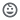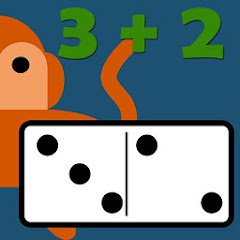100+Everyone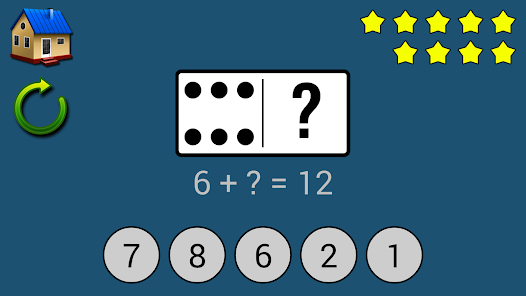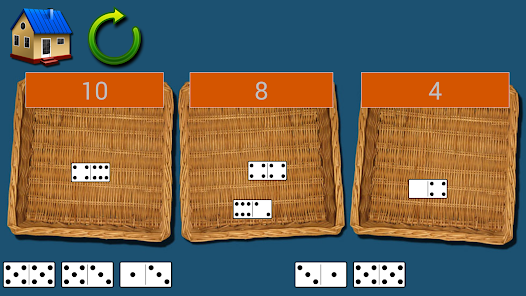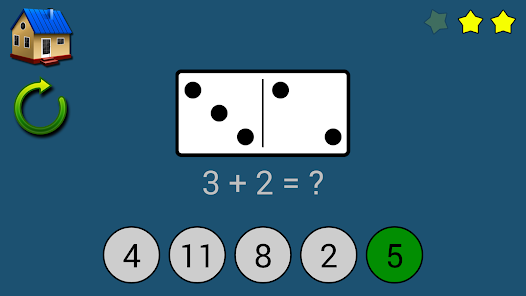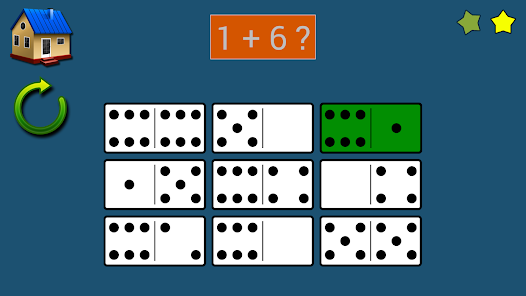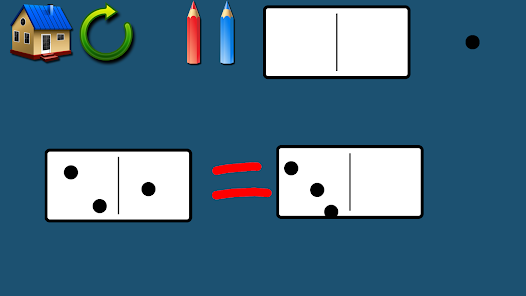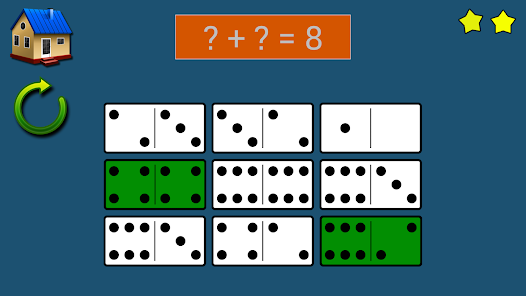Little Monkey Apps Dominoes Addition builds upon early subitising skills and extends this into early addition skills. Dominoes are used to teach basic sums, fill the gap sums and equal sums (equations)
using the familiar dot pattern found on dominoes.

Little Monkey Apps Dominoes Addition compliments, but does not replace the use of real dominoes for activities to encourage addition. The App is beneficial when using hands on equipment is not practical and to reinforce the activity in a different way to embed learning.

We encourage you as the educator to use real dominoes, print off blackline masters of blank dominoes and use sticky dots to have the children create their own dominoes.
Laminate them and play a game of dominoes.

Talk about the position of the dots and why the number 3 always looks a certain way on dominoes.

Use Little Monkey Apps Dominoes Addition as a blank canvas with all you need to use dominoes to teach. Two coloured pencils, eraser, dots and dominoes and a camera tool. Use this section with a small group of children or one on one. Not intended to be used by a student independently.

=Pick the domino=
Choose one domino from the nine that are shown to match the equation given. This ability aids students to recognise addition in familiar contexts and to partition a domino into two separate numbers, subitising (automatically recalling) a visual pattern of dots, not just of one number but of two numbers. Beginning from this task students can then come to see how moving a dot from one side to the other will not alter the answer but will change the numbers. When not using a tablet, this task can be completed se addition flash cards or 'real' dominoes.

Use the domino presented to complete the addition problem given. Students may need to count the dots beginning at one (count from zero) counting every individual dot, OR they may be more advanced and count on from one number i.e.. Knowing that one side is already four, so counting on five numbers to reach nine. More advanced students will use doubling or near doubles such as well four plus four is eight plus one is nine. Other students may use a visual strategy, manipulating the dots to visualise the answer. Without a tablet, this task can be completed as a worksheet with students writing the sum down.

=Sort the domino=
This task requires students to sort the dominoes into the correct basket. This task is asking students to come to realise that there is more than one way to reach the same answer. This task is about equations, that 4+4=2+6. This leads on to balance equations. In the classroom you might like to use buckets, placemats or whatever container you have in your classroom, label them with post it notes or stickers and put 'real' dominoes in each container. To demonstrate balance equations, use blocks and a balance scale to show that five red plus 2 green on one side is the same as four red plus 3 green. Play a game called target number, asking students to think of all the ways they can make a number i.e. 20, as students become more familiar with this, include subtraction and multiplication or with older children, brackets and negative numbers.

=Equal dominoes=
An introduction to equal sums (equations). Note that the answer to 1+5 is not only 6, but 3+3 is correct as well! Click on all of the dominoes that equal the number given.

=Complete the domino=
Solve the missing addend in the sum. Students can use the dots to assist them. A simple introduction to these often ignored problems. Discuss with your students how to work backwards using the dots or with counting skills. With advanced students you might want to discuss number fact partners, that is 5+2=7, 7-2=5 etc. In the classroom, this could also be a worksheet component.
Updated on
Nov 13, 2013

## Data safety

Safety starts with understanding how developers collect and share your data. Data privacy and security practices may vary based on your use, region, and age. The developer provided this information and may update it over time.No data shared with third parties